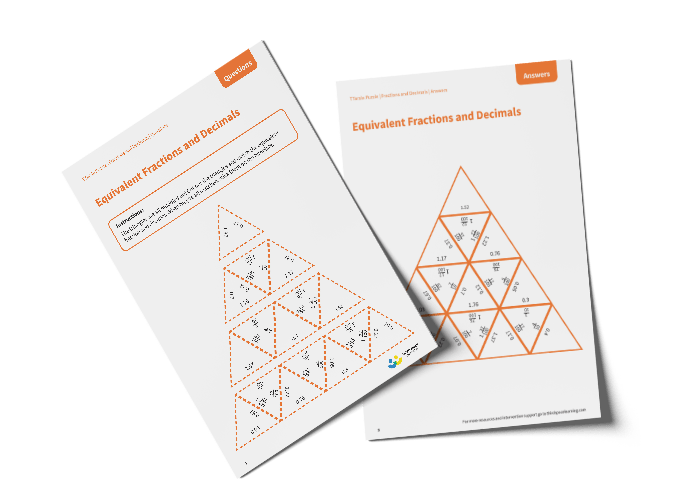# Tarsia Puzzle Equivalent Fractions and Decimals (Year 5)

The triangles in the tarsia puzzle have all been mixed up. In this equivalent fractions and decimals tarsia puzzle, pupils will need to match the fraction to the correct equivalent decimal to create one large triangle.

This Year 5 equivalent fractions and decimals tarsia puzzle worksheet contains:

• One question sheet
• One template sheet

Year 5

Fractions, Decimals